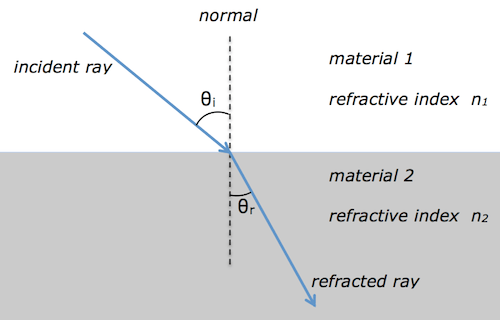## total internal reflection

Earlier this week, we used semi-circular perspex blocks to investigate total internal reflection.

I’ve put together a short video showing total internal reflection in a semicircular block and a perspex model of an optical fibre.

There are some nice ray diagrams explaining total internal reflection on BBC Bitesize.

Cyberphysics has some examples of how optical fibers are used and the youtube video below shows how they can be used by doctors to see inside a patient’s body.

## refraction, critical angle and total internal reflection

We met refraction during the National 5 course.  At Higher level, we are interested in the relationship between the angles of incidence θi and refraction θr.Snell’s law tells us that$n_1\sin \theta_i = n_2 \sin \theta_r$

Usually material 1 is air, and so$n_1 = 1$.  This simplifies Snell’s law to$\sin \theta_i = n \sin \theta_r$

where n is the absolute refractive index of material 2.  Since the refractive index is equal to the ratio of the ray’s speed v in materials 1 & 2 and also equal to the ratio of the wave’s wavelength λ in materials 1 & 2, we can show that$n = \displaystyle {{{\sin \theta_i} \over {\sin \theta_r}}} = \displaystyle {v_1 \over v_2} = \displaystyle {\lambda_1 \over \lambda_2}$

The critical angle$\theta_c$ and refractive index n are related by$\sin \theta_c = \displaystyle {1 \over n }$